# Intelligent Traffic Evaluation and Management System

Project ITEMS - An intelligent traffic evaluation, management and monitoring system with cloud data analysis.

IntermediateFull instructions provided20 hours284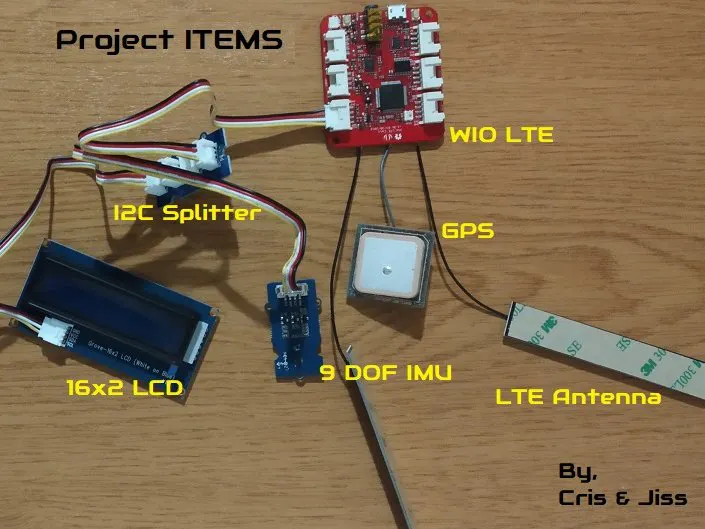## Things used in this project

### Hardware components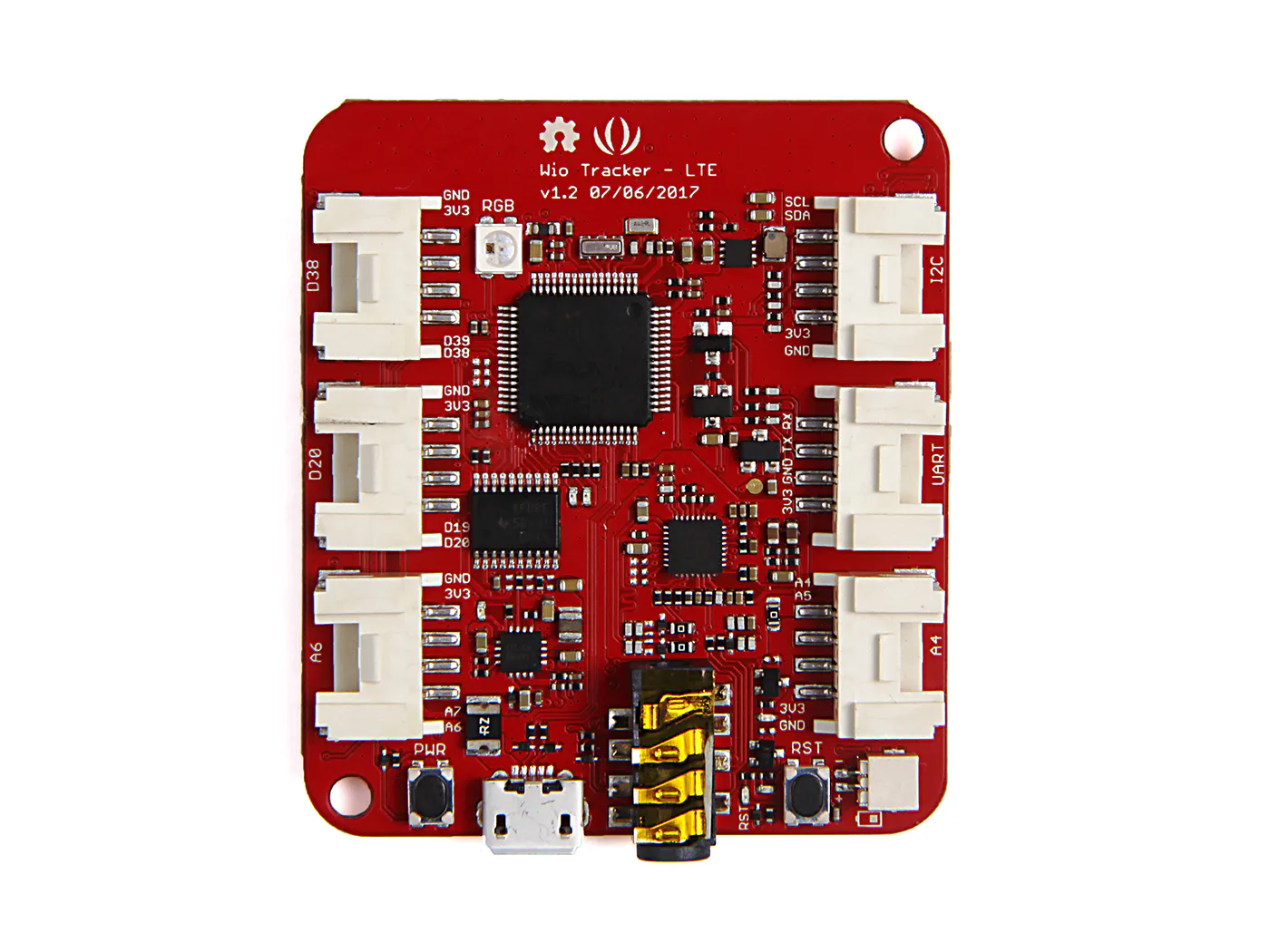Seeed Wio LTE US Version - 4G, Cat.1, GNSS, JavaScript(Espruino) Compatible
×1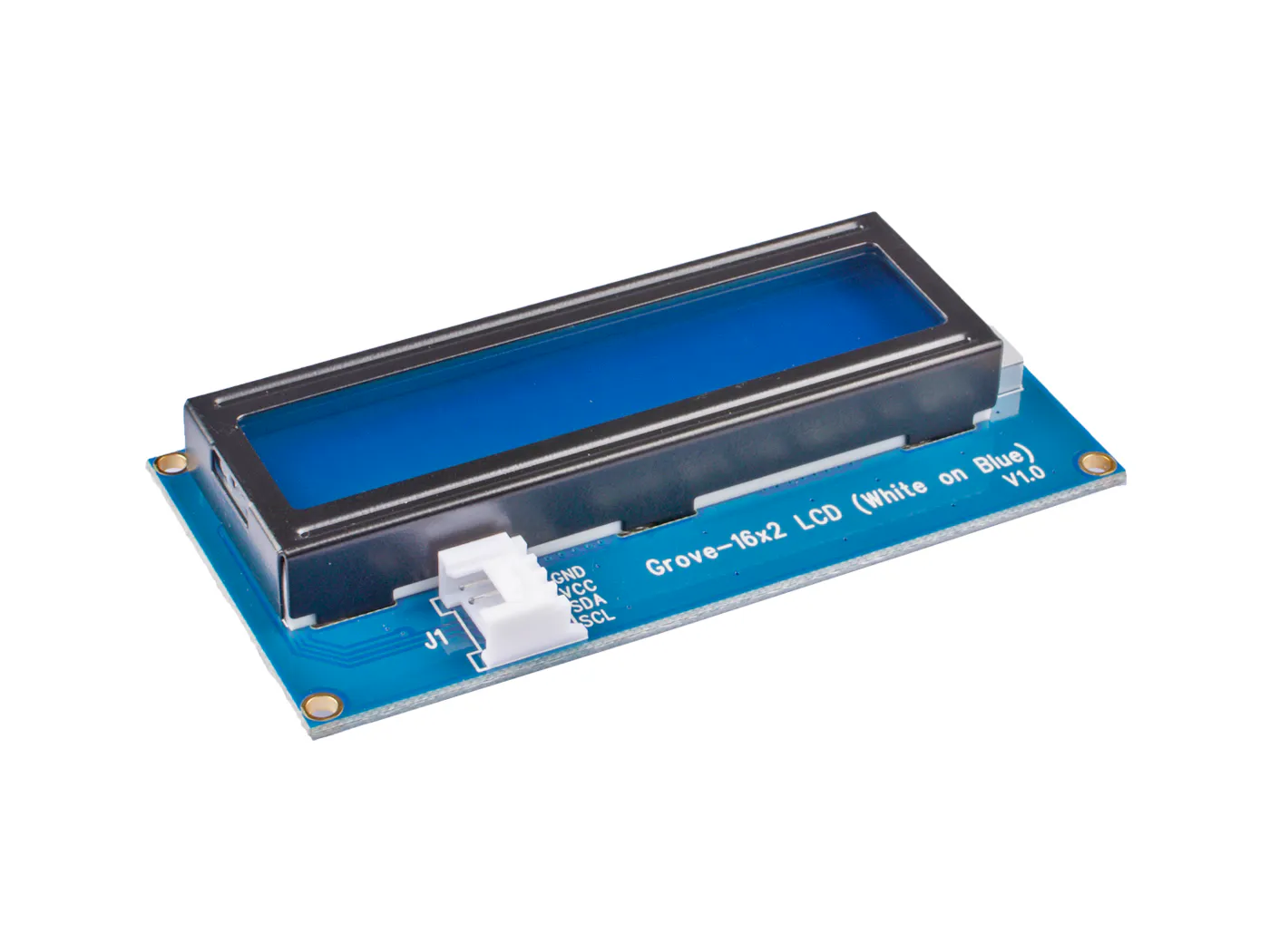Seeed Grove - 16 x 2 LCD (White on Blue)
×1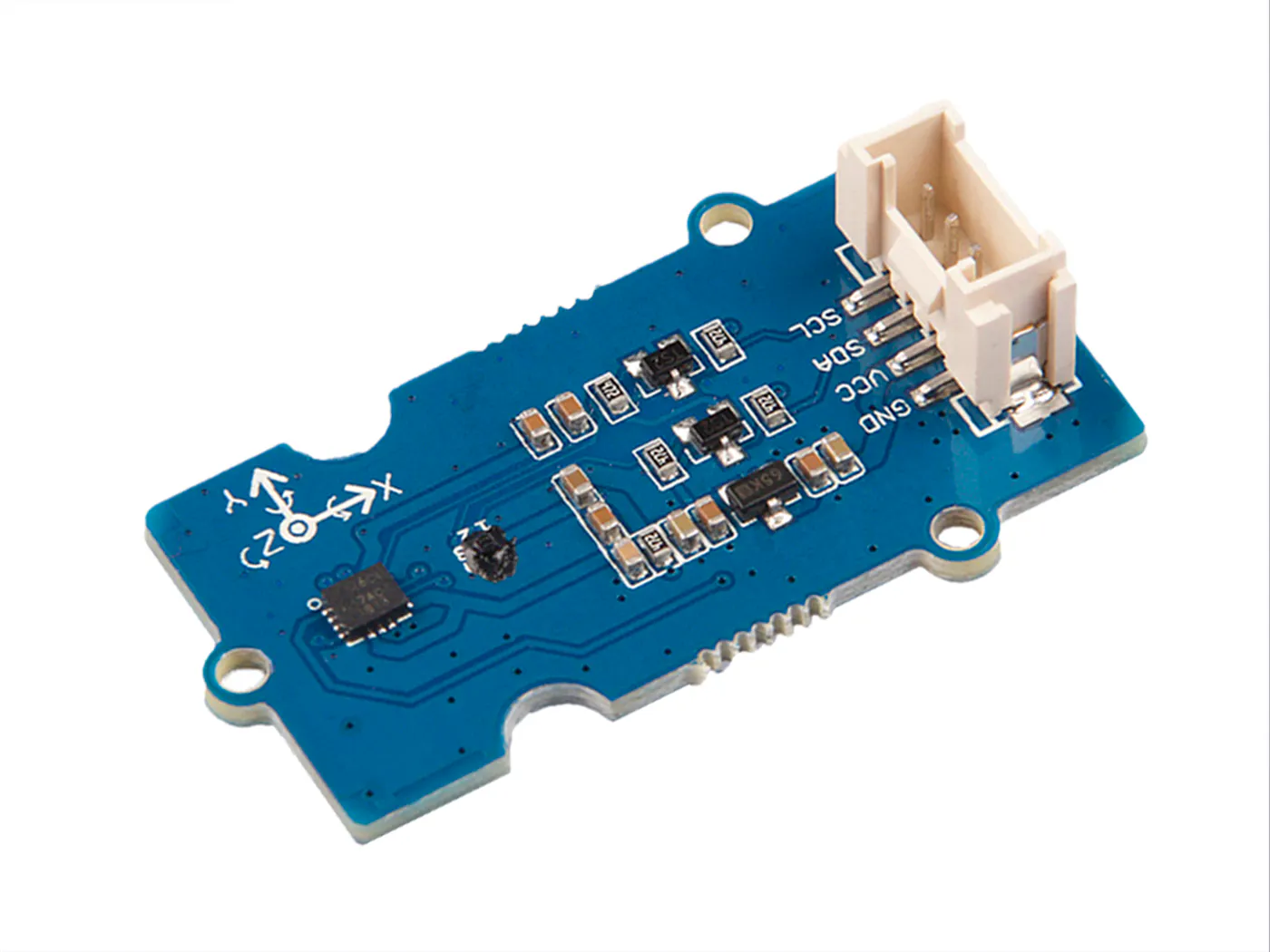Seeed Grove - IMU 9DOF (ICM20600+AK09918)
×1
×1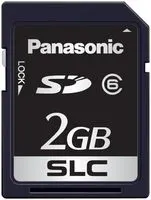Flash Memory Card, SD Card
×1

### Software apps and online servicesArduino IDE

## Code

### Project ITEMS

C/C++
Arduino sketch (given as C/C++ version for readability)
```//-------------------------------------------------------------------------------//
//-- PROJECT ITEMS                                                               //
//                                                                               //
//-- PROGRAM GOALS                                                               //
//-- An intelligent Traffic Evaluation, Management and Monitoring system         //
//-- with Cloud data analysis                                                    //
//                                                                               //
//-- Programmers: Cris Thomas, Jiss Joseph Thomas                                //
//-- References: WIO LTE                                                         //
//-- Contact: cris.thomas1@gmail.com, jissdeodates@gmail.com                     //
//-------------------------------------------------------------------------------//

#include "AK09918.h"
#include "ICM20600.h"
#include "gnss.h"
#include "rgb_lcd.h"
#include <Wire.h>
#include <Time.h>
#include <SD.h>

AK09918_err_type_t err;
int32_t x, y, z;
AK09918 ak09918;
ICM20600 icm20600(true);
int16_t acc_x, acc_y, acc_z;
int32_t offset_x, offset_y, offset_z;
double roll, pitch;
double declination_genova = +60.3;
rgb_lcd lcd;
const int chipSelect = 43;
File dataFile;

double vel, vel_x, vel_y, vel_z;
double lat, lon;
int start_t, now_t;
int sd_write = 0;

const int vehicle_id = 1;

void setup()
{
Wire.begin();
start_t = millis();

err = ak09918.initialize();
icm20600.initialize();
ak09918.switchMode(AK09918_POWER_DOWN);
ak09918.switchMode(AK09918_CONTINUOUS_100HZ);
while (err != AK09918_ERR_OK)
{
delay(100);
}
delay(2000);
calibrate(10000, &offset_x, &offset_y, &offset_z);

gnss.Power_On();
while(false == gnss.Check_If_Power_On()){
delay(1000);
}
if(!(gnss.open_GNSS())){
return;
}
delay(2000);

lcd.begin(16, 2);
delay(1000);

pinMode(SS, OUTPUT);
if (SD.begin(chipSelect))
{
sd_write = 1;
dataFile = SD.open("ITEMS_log.txt", FILE_WRITE);
dataFile.println("Project ITEMS");
dataFile.println("by Cris and Jiss");
dataFile.println("");
}

lcd.setCursor(0, 0);
lcd.print("Project ITEMS");
lcd.setCursor(0, 1);
lcd.print("By Cris and Jiss");

delay(1000);

lcd.setCursor(0, 0);
lcd.print("Vehicle ID -->");
lcd.setCursor(0, 1);
lcd.print(vehicle_id);
if(sd_write)
{
dataFile.println("Vehicle ID: " + vehicle_id);
}
}

void loop()
{
// get acceleration
acc_x = icm20600.getAccelerationX();
acc_y = icm20600.getAccelerationY();
acc_z = icm20600.getAccelerationZ();

ak09918.getData(&x, &y, &z);
x = x - offset_x;
y = y - offset_y;
z = z - offset_z;

roll = atan2((float)acc_y, (float)acc_z);
pitch = atan2(-(float)acc_x, sqrt((float)acc_y*acc_y+(float)acc_z*acc_z));
roll = roll*57.3;
pitch = pitch*57.3;
double Xheading = x * cos(pitch) + y * sin(roll) * sin(pitch) + z * cos(roll) * sin(pitch);
double Yheading = y * cos(roll) - z * sin(pitch);

delay(500);

// Finding velocity
now_t = millis();
vel_x = vel_x + (acc_x*(now_t - start_t));
vel_y = vel_y + (acc_x*(now_t - start_t));
vel_z = vel_z + (acc_x*(now_t - start_t));
vel = sqrt((vel_x^2) + (vel_y^2));

lcd.setCursor(0, 0);
lcd.print("Velocity -->");
lcd.setCursor(0, 1);
lcd.print(vel + " m/s");
if(sd_write)
{
dataFile.println("Velocity: " + vel);
}

if(gnss.getCoordinate())
{
lon = gnss.longitude;
lat = gnss.latitude;

lcd.setCursor(0, 0);
lcd.print("GNSS : Lat -->");
lcd.setCursor(0, 1);
lcd.print(lat);
if(sd_write)
{
dataFile.println("Lat: " + lat);
}

lcd.setCursor(0, 0);
lcd.print("GNSS : Long -->");
lcd.setCursor(0, 1);
lcd.print(lon);
if(sd_write)
{
dataFile.println("Long: " + lon);
}
}

}

void calibrate(uint32_t timeout, int32_t *offsetx, int32_t *offsety, int32_t*offsetz)
{
int32_t value_x_min = 0;
int32_t value_x_max = 0;
int32_t value_y_min = 0;
int32_t value_y_max = 0;
int32_t value_z_min = 0;
int32_t value_z_max = 0;
uint32_t timeStart = 0;

ak09918.getData(&x, &y, &z);

value_x_min = x;
value_x_max = x;
value_y_min = y;
value_y_max = y;
value_z_min = z;
value_z_max = z;
delay(100);

timeStart = millis();

while((millis() - timeStart) < timeout)
{
ak09918.getData(&x, &y, &z);

if(value_x_min > x)
{
value_x_min = x;
}
else if(value_x_max < x)
{
value_x_max = x;
}

if(value_y_min > y)
{
value_y_min = y;
}
else if(value_y_max < y)
{
value_y_max = y;
}

if(value_z_min > z)
{
value_z_min = z;
}
else if(value_z_max < z)
{
value_z_max = z;
}

Serial.print(".");
delay(100);

}

*offsetx = value_x_min + (value_x_max - value_x_min)/2;
*offsety = value_y_min + (value_y_max - value_y_min)/2;
*offsetz = value_z_min + (value_z_max - value_z_min)/2;
}
```

## Credits

### Cris Thomas

8 projects • 43 followers
Electronics and Aerospace engineer with a dedicated history in Research and Development

### Jiss Joseph Thomas

6 projects • 36 followers
Co-Researcher Trainee (Artificial Intelligence) at Creopedia Business Intelligence Pvt.Ltd, Kochi,